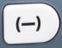# NegativesBasics
Positive = any number greater than zero
Positive numbers are not usually accompanied by a preceding + sign.$\bf\displaystyle\frac{1}{2}$,$\bf\displaystyle1\frac{1}{2}$,    37,    215,    etc.

Negative = any number less than zero
Negative numbers are usually accompanied by a preceding – sign.
-215,    -37,    –$\bf\displaystyle1\frac{1}{2}$,    –$\bf\displaystyle\frac{1}{2}$,    etc.

is the negative sign.is the negative command.

Signs
Two consecutive negative signs produce a positive sign.
– -3 = +3 = 3

A negative sign adjacent to a positive sign produces a negative sign.
– +3 = -3
+ -3 = -3

The sum of a negative and a positive can be negative, positive, or zero.
-5 + 3 = -2
3 + -5 = -2
-5 + 9 = 4
9 + -5 = 4
-6 + 6 = 0
6 + -6 = 0

Input Display Commentblinker clears screen5 + 3 5+3–2 Answerblinker clears screen
3 +5 3+5–2 Answerblinker clears screen5 + 9 –5+94 Answerblinker clears screen
9 +5 9+54 Answerblinker clears screen6 + 6 –6 + 60 Answerblinker clears screen
6 +6 6 + –60 Answer
###### Negatives

Subtraction
The difference between a negative and a positive is a negative.
-5 – 3 = -8

The difference between a positive and a negative can be positive or zero.
3 – -5 = 8
3 – -3 = 0

The difference between a negative and a negative can be negative, positive, or zero.
-5 – -3 = -2
-3 – -5 = 2
-6 – -6 = 0

Input Display Commentblinker clears screen5 – 3 5-3–8 Answerblinker clears screen
3 –5 3-58 Answerblinker clears screen
3 –3 3-30 Answerblinker clears screen5 –3 5-3–2 Answerblinker clears screen3 –5 3-52 Answerblinker clears screen6 –6 6-60 Answer
###### Negatives

Multiplication
Negative × Positive = Negative
Positive × Negative = Negative
-5 × 3 = -15
5 × -3 = -15

Negative × Negative = Positive
-5 × -3 = 15
-3 × -5 = 15

Negative × Zero = Zero
Zero × Negative = Zero
-6 × 0 = 0
0 × -6 = 0

Input Display Commentblinker clears screen5 × 3 5*315 Answerblinker clears screen
5 ×3 5*315 Answerblinker clears screen5 ×3 5*315 Answerblinker clears screen3 ×5 3*515 Answerblinker clears screen6 × 0 6*00 Answerblinker clears screen
0 ×6 0*60 Answer
###### Negatives

Division
Negative ÷ Positive = Negative
Positive ÷ Negative = Negative
-15 ÷ 3 = -5
15 ÷ -3 = -5

Negative ÷ Negative = Positive
-15 ÷ -3 = 5
-15 ÷ -5 = 3

Zero ÷ Negative = Zero
Negative ÷ Zero = Undefined
0 ÷ -6 = 0
-6 ÷ 0 = Undefined

Input Display Commentblinker clears screen15 ÷ 3 15÷35 Answerblinker clears screen
15 ÷3 15÷35 Answerblinker clears screen15 ÷3 15÷35 Answerblinker clears screen15 ÷5 15÷53 Answerblinker clears screen
0 ÷6 60 Answerblinker clears screen6 ÷ 0 6÷0DIVIDE BY 0
Error
###### Negatives

Practice – Questions
1.  What is -7 + 15?

2.  What is 6 + -11?

3.  What is -13 – 8?

4.  What is -4 – -9?

5.  What is -12 × 13?

6.  What is -14 × -15?

7.  What is -169 ÷ 13?

8.  What is -144 ÷ -12?

9.  What is -8 + -4 × -12 ÷ -6?

10.  What is -8 – -4 × -15 ÷ -6?

1.  8

2.  -5

3.  -21

4.  5

5.  -156

6.  210

7.  -13

8.  12

9.  -16

10.  2